# Help: Collision detection for moving objects

Ok. With my extremely limited coding knowledge, I've gotten far further than I thought I'd be able to, but I've been bashing my head against this math on and off for a couple of weeks now and it's a complete blocker for continued dev on my game, so I'm appealing for some helpI've been avoiding asking here due in part to the amount of code I might need to paste, and being a bit embarrassed about the likely terrible code quality, but here goes.

The collision detection in my game is working great for a moving ball colliding with static bricks (pictured below).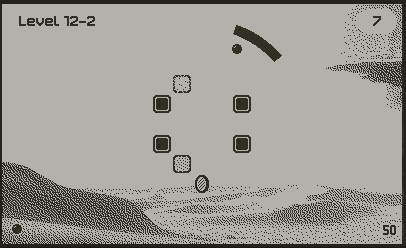I'm using the Playdate's sprite collision library, that sits within my ball's update function, below:

``````function Ball:update(paddleX, paddleY, isMagnetActive)
-- stick ball to paddle if it hasn't been fired yet
self:updateUnfiredPosition()

-- calculate anticipated position based on direction, speed, and angular velocity (if any)
self.currentDirection = atan2(self.velocityY, self.velocityX)
self.currentDirection = self.currentDirection + self.angularVelocity
self.velocityX = self.speed * cos(self.currentDirection)
self.velocityY = self.speed * sin(self.currentDirection)
self.targetX = self.x + self.velocityX * gameSpeed
self.targetY = self.y + self.velocityY * gameSpeed

-- reduce angular velocity(spin) a little each frame
self.angularVelocity = self.angularVelocity * angularVelocityDecay

-- check for collisions
local actualX, actualY, collisions, length = self:moveWithCollisions(self.targetX, self.targetY)

-- handle collision rebounds (poorly?)
for i = 1, length do
local collision = collisions[i]

-- Only care about these 3 types of objects for now
if collision.other.type == "brick" or collision.other.type == "sand" or collision.other.type == "ice" then
-- trigger hit function that handles vfx and sounds
collision.other:hit(self, collision.normal)  -- Passing collision normal here

-- collision normal
local normalX = collision.normal.x
local normalY = collision.normal.y

-- calculate brick velocity
local brickVelX = collision.other.velocityX or 0
local brickVelY = collision.other.velocityY or 0

print("Brick vel", brickVelX, brickVelY, "Collision normal", normalX, normalY)
print("Ball x/y:", self.x, self.y, "Actual x/y:", actualX, actualY, "Target x/y", self.targetX, self.targetY)

-- Only use the brick's velocity component perpendicular to the collision normal
if normalX ~= 0 then
self.velocityX = -self.velocityX -- brickVelX * normalX
actualX = actualX + brickVelX
end
if normalY ~= 0 then
self.velocityY = -self.velocityY -- brickVelY * normalY
actualY = actualY + brickVelY
end

break
end
end

-- curve trajectory towards paddle when magnet is active
if isMagnetActive then
end

-- set actual position based on the above
self.x = actualX
self.y = actualY

if debug then
self:trackHistory()
end
end

function Ball:collisionResponse(other)
return "bounce"
end
``````

The moving bricks are handled by an update function that runs every frame (warning, this may be rough):

``````function updatePositions(objList)
for _, obj in ipairs(objList) do
if obj.path and obj.currentPathPoint and obj.currentLerpValue then
if obj.waitTime and obj.waitTime > 0 then
obj.waitTime = obj.waitTime - gameSpeed -- Subtract gameSpeed from waitTime
else
local prevPoint = obj.path[obj.currentPathPoint]
local nextPoint = obj.path[obj.currentPathPoint % #obj.path + 1]

obj.currentLerpValue = obj.currentLerpValue + (obj.speed[obj.currentPathPoint] * gameSpeed)
obj.currentLerpValue = clamp(obj.currentLerpValue, 0, 1)

local x = lerp(prevPoint, nextPoint, obj.currentLerpValue)
local y = lerp(prevPoint, nextPoint, obj.currentLerpValue)

x = round(x)
y = round(y)

if obj.prevX and obj.prevY then
obj.velocityX = (x - obj.prevX) --/ gameSpeed
obj.velocityY = (y - obj.prevY) --/ gameSpeed
end

obj.prevX = x
obj.prevY = y

if obj.type == "coin" then
obj:moveTo(x, y)
else
obj:moveTo(x, y)
end

if obj.currentLerpValue >= 1 then
obj.currentLerpValue = 0
obj.currentPathPoint = obj.currentPathPoint % #obj.path + 1
if obj.path[obj.currentPathPoint] then
obj.waitTime = obj.path[obj.currentPathPoint] -- waitTime now directly set in seconds
else
obj.waitTime = 0
end

-- Here, we explicitly set the object's velocity to zero when it reaches its target position.
obj.velocityX = 0
obj.velocityY = 0
end
end
end
end
end
``````

So I'm having two problems, likely related:

When a brick is moving quite fast, and a collision occurs, the ball tunnels through the brick in an unwanted way, as shown here: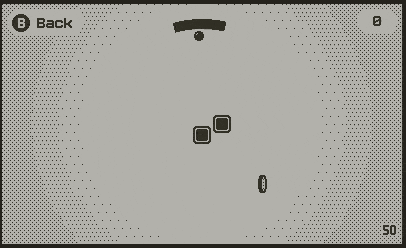When the brick is moving slower (which it does on the down motion), the problem doesn't occur, presumably as the ball's speed is greater than the brick, causing it not to trigger multiple collisions: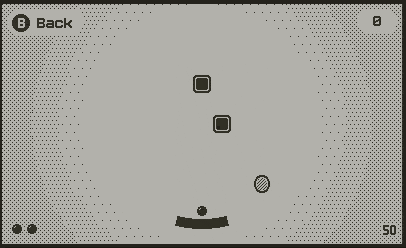I know the collision detection in the Playdate SDK handles tunnelling, and when I comment out my collision handling code, there's no bounce, but no tunnelling either, so the problems are squarely with my code.

One of the things I thought might be an issue, is that I'm moving the brick with moveTo() rather than moveWithCollisions(). (hence the weird redundant if statement in updatePositions, ignore that please) Problem was when I was using moveWithCollisions for the brick, it would cause the brick to fail to get to its actual end position much of the time. I want the brick's position to be completely unaffected by the ball. Unsure if this is the right approach or not.

So that's problem 1.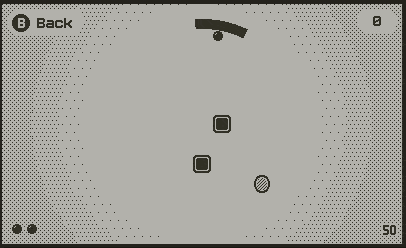Problem 2 is that I'm trying to get the brick's impact to affect the velocity/direction of the brick, which is most noticeable when the hit is perpendicular to the ball's direction: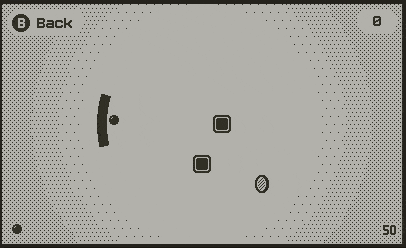The green here is sorta what I expect to happen, the red is what actually happens.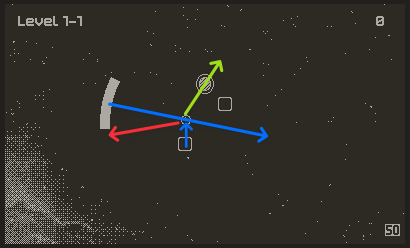Not expecting a working solution (though that'd be lovely), but if anyone can point me in a direction even, or point out clear and obvious flaws, that'd be fantastic. Thank you all so much.

Based on a suggestion from the Discord, I changed the brick back to using moveWithCollisions(), and set the brick response to be overlap to avoid the position being affected by the ball. This worked in that the ball doesn't affect the brick's position, but the ball behaviour seems to be the same as it is here for both problems still.

If I'm reading your code right, you're simply reversing the velocity in each axis based on the normal. As you've discovered, this will not result in the desired ball velocity after the collision. You'll need to do some calculations around conservation of momentum. This means giving the ball and the block a mass, and calculating the before and after for the collision. There's plenty of sites out there that'll go through it, usually to do with circle-circle collisions but if you have an accurate normal from the collision system then it should plug in fine. Here's a pretty good one: Collisions of Point Masses in Two Dimensions | Physics

As for your problem with fast moving objects, most physics engines solve this by stepping the whole system in multiple much shorter time periods between each frame, or by moving the item iteratively in small steps across the whole movement. If moveWithCollisions solves this for you, great. If not, you'll have to set a speed threshold above which you break down the velocity into smaller parts and move the item, then test collisions, then move it again, test again etc.

1 Like

In your case, you probably want the block to be moving at the same velocity that it was before the collision, so you already know the resulting velocity for the block. This should make the calculation a lot easier. You also might be getting strange normals out if your block is moving too fast, make sure it works with slow moving stuff first.

I'm interested int he normal you are getting actually, definitely consider rendering it to the screen so you can see it's correct

Thanks for the replies! Yeah so I will definitely try to visualize the normals. I think for the most part the first collision has the expected normals, but when the brick is moving faster than the ball, it registers a second collision that's sometimes over the half way point of the brick, which I think causes the opposite normal to be returned.

I've definitely got versions of it working (with better reflection angle calculations) when the brick is moving slowly, just due to the fact that the ball moves out of the way fast enough and doesn't register 2nd quick collisions or tunnel at all.

I think there might be an issue causing some weirdness at the end of the update where I'm setting self.x and self.y to actualX and actualY, as this means I'm effectively moving the position twice per frame (once without collisions?) When I remove this it's still broken, but broken in the same way, so that code doesn't appear to be helping.

I think part of the reason this code is so hard to work with is that the way the ball and brick velocities are calculated aren't the same, so it's hard to do something like add the brick's velocity to the ball to try and have it avoid the brick next frame.

The part of this whole thing that indicates that I don't really understand what's going on under the hood, is when I comment out all the collision for loop, and it doesn't tunnel or bounce even at really high speeds, it just slides off the brick (even though the response is set to bounce).

Thinking about this more, you probably don't actually want to conserve momentum if the block's velocity is unaltered. My physics is so rusty ha. Probably you'd calculate the velocities as if the block were not a fixed velocity, then disregard the result and set the block's velocity after.

If you want I could find some time to take a look. Feel free to DM me.

1 Like

So I dug myself out of my hole, and am trying to work through this problem again. The core of it seems to be that the collision normal from the collision library isn't returning the value I expect (and I'm not sure why). Here's some quick debugging where I'm drawing a line along the collision normal. You can see for static bricks, everything's working as expected, but with the moving bricks, stuff's getting real weird.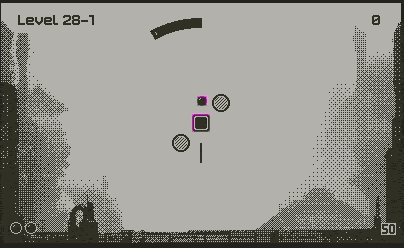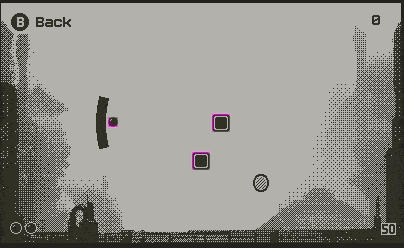I've decided if I can get the bounce angles working correctly, I'm just going to limit the max brick movement speed to a speed that's a tiny bit less than the ball, so hopefully I can prevent tunnelling in most situations. Not ideal, but a tradeoff I'm willing to make to unblock myself at this point.

1 Like